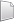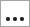# HS-1035: Optimization Study using an Excel Spreadsheet

Learn how to use couple HyperStudy with a spreadsheet, identify input variables and output responses, and run an Optimization study.

Before you begin, copy the model files used in this tutorial from <hst.zip>/HS-1035/ to your working directory.
Problem formulation
• Find the cross-sectional dimension's width and height in mm.
• Minimize the beam volume such that the tip deflection < 0.53 mm.

When you create an Excel spreadsheet model, it is important that the spreadsheet is formatted correctly. A variable's value and label can be formatted in two consecutive rows or two consecutive columns. Variable labels should only contain English characters, or a combination of English characters and numbers. If a label is not created for a variable, HyperStudy will assign one by default.

1. In Excel, open the hst_tut_1035(1070)_spreadsheet.xls file.
2. Review the information, and locate the columns that contain the input variables and output responses.

## Perform the Study Setup

1. Start HyperStudy.
2. Start a new study in the following ways:
• From the menu bar, click File > New.
• On the ribbon, click.
3. In the Add Study dialog, enter a study name, select a location for the study, and click OK.
4. Go to the Define Models step.
5. Add a Spreadsheet model by dragging-and-dropping the hst_spreadsheet_integration_example file from the Directory into the work area.
The Resource and Solver input file fields become populated. The Solver input file field displays hst_input.hstp, this is the name of the solver input file HyperStudy writes during an evaluation.
6. Click Import Variables.
1. In the Excel dialog, click Yes to begin selecting input variables.
2. In the spreadsheet, select the cells that contain the input variable's labels and values.
3. In the Excel dialog, click OK.
4. Click Cancel to stop selecting input variables.
1. In the Excel dialog, click Yes to begin selecting output responses.
2. In the spreadsheet, select the cells that contain the output response's labels and values.
3. In the Excel dialog, click OK.
4. Click Cancel to stop selecting output responses.
Two input variables and two output responses are imported from the hst_tut_1035(1070)_spreadsheet.xls spreadsheet.
9. Go to the Define Input Variables step.
10. Review the input variable's lower and upper bound ranges.

## Perform Nominal Run

1. Go to the Test Models step.
2. Click Run Definition.
An approaches/setup_1-def/ directory is created inside the study Directory. The approaches/setup_1-def/run__00001/m_1 directory contains the input file, which is the result of the nominal run.

## Review Output Responses

1. Go to the Define Output Responses step.
2. Review the output responses imported into the study.
The output responses were extracted from the hst_output.hstp file, which HyperStudy created for each run.

## Run Optimization

1. In the Explorer, right-click and select Add from the context menu.
2. In the Add dialog, select Optimization and click OK.
2. Go to the Optimization > Definition > Define Input Variables step.
3. Apply a set range of +17% to both input variable's lower and upper bounds.
1. In the Lower Bound column of both input variables, click.
2. In the Percent field, enter +17.
3. Click the +/- button.
4. Click Apply.
4. Go to the Optimization > Definition > Define Output Responses step.
5. Click the Objectives/Constraints - Goals tab.
6. Assign an objective to the Beam Volume (m^3) output response.
2. In the Apply On column, select Beam Volume (m^3).
3. In the Type column, select Minimize.
7. Assign a constraint to the Deflection at the tip (mm) output response.
2. In the Apply On column, select Deflection at the tip (mm).
3. In the Type column, select Constraint.
4. In column 1, select <= (less than or equal to).
5. In column 2, enter 0.53.
8. Go to the Optimization > Specifications step.
9. In the work area, set the Mode to Adaptive Response Surface Method (ARSM).
Note: Only the methods that are valid for the problem formulation are enabled.
10. Click Apply.
11. Go to the Evaluate step.